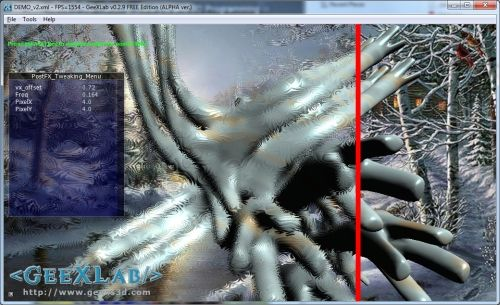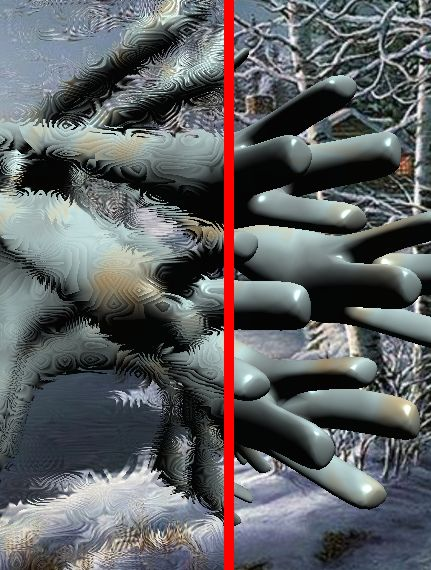# [Shader Library] Frosted Glass Post Processing Shader (GLSL)Article Index

Today, to celebrate these winter days, I propose you a GLSL-based post processing filter that looks like viewing through an ice-frosted glass. I found two implementations of a frosted glass effect and both have been tested and converted to GeeXLab.

More shaders are available in the Shader Library.

## 1 – Download

You can grab the GLSL shaders and GeeXLab demos here:
[download#196#image]
Left-click to download (right-click disabled)

These demos require GeeXLab 0.2.9 and are not based on Python so you can use the version of GeeXLab without Python.

Unzip the source code somewhere, start GeeXLab and drop the file DEMO.xml in GeeXLab.

The 3D model used in both demos has been created with the Qoob modeller. GeeXLab since version 0.2.7 supports the loading of Qoob meshes. More information about Qoob can be found here.

## 2 – Frosted Glass Effect: First GLSL shaderLanguage: OpenGL 2 – GLSL

Type: Post processing filter.

Inputs

• sceneTex (sampler2D): the final scene image.
• NoiseTex (sampler2D): noise texture.
• vx_offset (float): x position of the vertical red line
• PixelX (float): effect param, default value: 2.0
• PixelY (float): effect param, default value: 2.0
• Freq (float): effect param, default value: 0.0115

Ouput: color buffer

```[Vertex_Shader]
void main(void)
{
gl_Position = ftransform();
gl_TexCoord = gl_MultiTexCoord0;
}
[Pixel_Shader]
uniform sampler2D sceneTex; // 0
uniform sampler2D NoiseTex; // 1
uniform float vx_offset;
uniform float PixelX = 2.0;
uniform float PixelY = 2.0;
uniform float Freq = 0.115;
uniform float rt_w; // GeeXLab built-in
uniform float rt_h; // GeeXLab built-in

vec4 spline(float x, vec4 c1, vec4 c2, vec4 c3, vec4 c4, \
vec4 c5, vec4 c6, vec4 c7, vec4 c8, vec4 c9)
{
float w1, w2, w3, w4, w5, w6, w7, w8, w9;
w1 = 0.0;
w2 = 0.0;
w3 = 0.0;
w4 = 0.0;
w5 = 0.0;
w6 = 0.0;
w7 = 0.0;
w8 = 0.0;
w9 = 0.0;
float tmp = x * 8.0;
if (tmp<=1.0) {
w1 = 1.0 - tmp;
w2 = tmp;
}
else if (tmp<=2.0) {
tmp = tmp - 1.0;
w2 = 1.0 - tmp;
w3 = tmp;
}
else if (tmp<=3.0) {
tmp = tmp - 2.0;
w3 = 1.0-tmp;
w4 = tmp;
}
else if (tmp<=4.0) {
tmp = tmp - 3.0;
w4 = 1.0-tmp;
w5 = tmp;
}
else if (tmp<=5.0) {
tmp = tmp - 4.0;
w5 = 1.0-tmp;
w6 = tmp;
}
else if (tmp<=6.0) {
tmp = tmp - 5.0;
w6 = 1.0-tmp;
w7 = tmp;
}
else if (tmp<=7.0) {
tmp = tmp - 6.0;
w7 = 1.0 - tmp;
w8 = tmp;
}
else
{
//tmp = saturate(tmp - 7.0);
// http://www.ozone3d.net/blogs/lab/20080709/saturate-function-in-glsl/
tmp = clamp(tmp - 7.0, 0.0, 1.0);
w8 = 1.0-tmp;
w9 = tmp;
}
return w1*c1 + w2*c2 + w3*c3 + w4*c4 + w5*c5 + w6*c6 + w7*c7 + \
w8*c8 + w9*c9;
}

vec3 NOISE2D(vec2 p)
{ return texture2D(NoiseTex,p).xyz; }

void main()
{
vec2 uv = gl_TexCoord.xy;
vec3 tc = vec3(1.0, 0.0, 0.0);

if (uv.x < (vx_offset-0.005))
{
float DeltaX = PixelX / rt_w;
float DeltaY = PixelY / rt_h;
vec2 ox = vec2(DeltaX,0.0);
vec2 oy = vec2(0.0,DeltaY);
vec2 PP = uv - oy;
vec4 C00 = texture2D(sceneTex,PP - ox);
vec4 C01 = texture2D(sceneTex,PP);
vec4 C02 = texture2D(sceneTex,PP + ox);
PP = uv;
vec4 C10 = texture2D(sceneTex,PP - ox);
vec4 C11 = texture2D(sceneTex,PP);
vec4 C12 = texture2D(sceneTex,PP + ox);
PP = uv + oy;
vec4 C20 = texture2D(sceneTex,PP - ox);
vec4 C21 = texture2D(sceneTex,PP);
vec4 C22 = texture2D(sceneTex,PP + ox);

float n = NOISE2D(Freq*uv).x;
n = mod(n, 0.111111)/0.111111;
vec4 result = spline(n,C00,C01,C02,C10,C11,C12,C20,C21,C22);
tc = result.rgb;
}
else if (uv.x>=(vx_offset+0.005))
{
tc = texture2D(sceneTex, uv).rgb;
}

gl_FragColor = vec4(tc, 1.0);
}
```

Credits
This frosted glass shader is a modified version for GeeXLab of NVIDIA’s ice-frosted glass HLSL shader.

Second GLSL shader: next page please!

Article Index